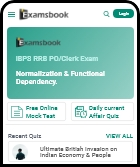1163 0

• 1
58 l
• 2
65 l
• 3
50 l
• 4
62 l
• Workspace

## Answer : 3. "50 l" Explanation :Answer: C) 50 l Explanation: Let the tin contain 5x litres of liquids 5x - 45 x 455x - 45 x 15 + 45 = 25 => 5(4x - 36) = 2(x + 36) => 20x - 180 = 2x + 72 => x = 14 litres Hence, the initial quantity of mixture = 70l Quantity of liquid B = 70 - 45 x 15 + 45  = 50 litres.

### Are you sure